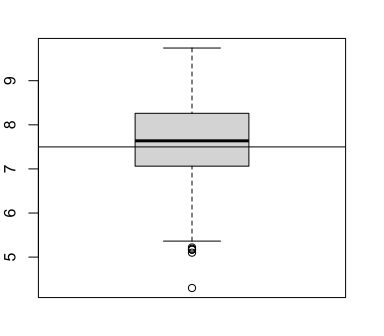# Statistiek I

## Nonparametric tests

Martijn Wieling
University of Groningen

## Last lecture

• Three variants of the $$t$$-test
• How to calculate the effect size (Cohen's $$d$$)
• How to report results of a statistical test

## This lecture

• Nonparametric tests:
• Mann-Whitney U test: alternative to independent samples $$t$$-test
• Wilcoxon signed-rank test: alternative to paired and single sample $$t$$-test
• Sign test: alternative to Wilcoxon signed-rank test
• Reporting statistical analyses (again)

## Nonparametric tests

• Nonparametric tests do not assume an underlying distribution (of the data) and therefore have no parameters
• In contrast to e.g., $$N(0,1)$$ and $$t(18)$$
• Nonparametric tests are applied when the distribution is unknown or the required assumptions of the parametric test are violated
• They can also be applied to data assumed to be normally distributed
• Often best option for nonnumeric data (next lecture: $$\chi^2$$)
• Less sensitive than parametric tests (i.e. less power)!

## Popular nonparametric tests

• Mann-Whitney U test: alternative to independent samples $$t$$-test
• When data normally distributed: 95% of power of $$t$$-test
• Wilcoxon signed-rank test: alternative to paired $$t$$-test
• Requirement: distribution symmetrical
• When data normally distributed: 95% of power of $$t$$-test
• Sign test: alternative to Wilcoxon signed-rank test when data not symmetrical

## Mann-Whitney U test

• Alternative to independent samples $$t$$-test (i.e. comparing two indep. samples)
• Applicable to ordinal data (there is an ordering: no exact scale) and num. data
• Also when $$n \leq$$ 30 and data in (at least) one group not normally distributed
• $$H_0$$: $$P(X > Y) = P(Y > X)$$, $$H_a$$: $$P(X > Y) \neq P(Y > X)$$
• If distributions of samples the same, this also means:
$$H_0$$: medians of both groups equal, $$H_a$$: medians of both groups differ
• Frequently applied to Likert data: on a scale from 1 (easiest) to 5 (hardest) ...
• (Identical to: Wilcoxon's rank sum test)

## Mann-Whitney U test: idea

• Idea: combine the two sets of values, order them from low to high and count how often the items in one set come after items in the other set
• Group A: (2, 4, 6, 10, 20), Group B: (8, 12, 14, 16, 18)
• Ordered: A A A B A B B B B A (values: 2, 4, 6, 8, 10, 12, 14, 16, 18, 20)
• $$U_A = 0 + 0 + 0 + 1 + 5 = 6$$  (A)
• $$U_B = 3 + 4 + 4 + 4 + 4 = 19$$  (B)
• Mann-Whitney U $$= min(U_A,U_B) = 6$$
• The lower U, the more likely to be significantly different
• $$p$$-value: obtained by assessing where $$U$$ is located in the distribution of all possible $$U$$-values for given sample sizes $$n_A$$ and $$n_B$$
• Distribution of all $$U$$-values resembles a normal distribution (for larger $$n$$)

## Distribution of $$U$$-values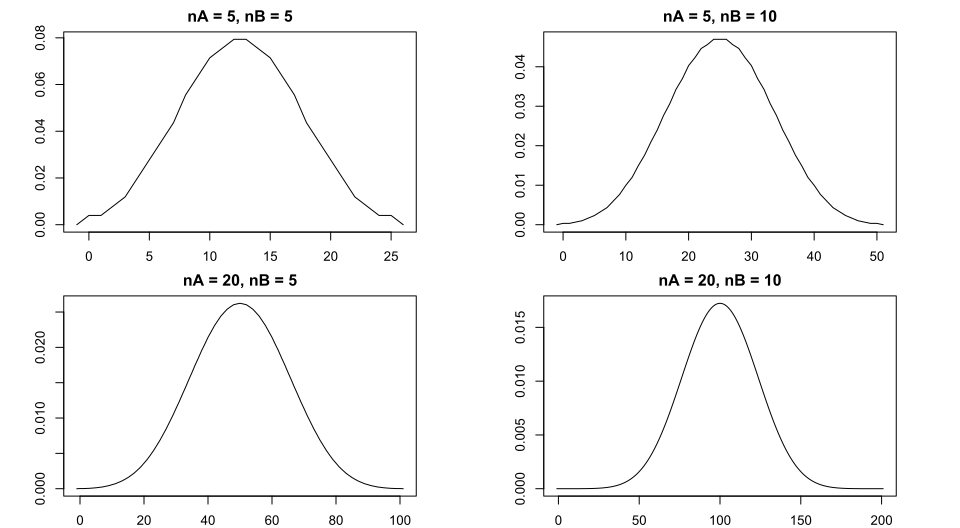## Mann-Whitney U test: additional information

• In R: wilcox.test()
• Identical usage as t.test()
• With Mann-Whitney U test: data is converted to ranks
• Actual values are ignored: loss of information!

## Example: tongue difference between /θ/ and /t/## Study: native vs. non-native English

• Research question: Do native English speakers show a stronger distinction of /t/ from /θ/ ("th") with their tongue than non-native (Dutch) speakers of English?
• Hypothesis: The tongue position difference between /t/ and /θ/ is larger for English native speakers than for non-native Dutch speakers of English
• $$H_0$$: same frontal /t/-/θ /position difference for Dutch and English speakers
• $$H_a$$: larger frontal /t/-/θ position difference for English (versus Dutch) speakers

## Study: native vs. non-native English

• Data: 22 English and 19 Dutch participants who pronounced 10 minimal pairs
/t/:/θ/ while connected to the articulography device:
• 'fate'-'faith', 'forth'-'fort', 'kit'-'kith', 'mitt'-'myth', 'tent'-'tenth'
• 'tank'-'thank', 'team'-'theme', 'tick'-'thick', 'ties'-'thighs', 'tongs'-'thongs'
• For each speaker, we calculated the average difference in frontal tongue position between /t/-words and /θ/-words

## Data visualization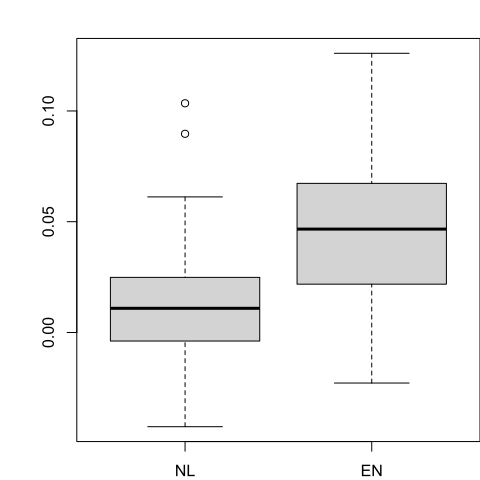## Distributions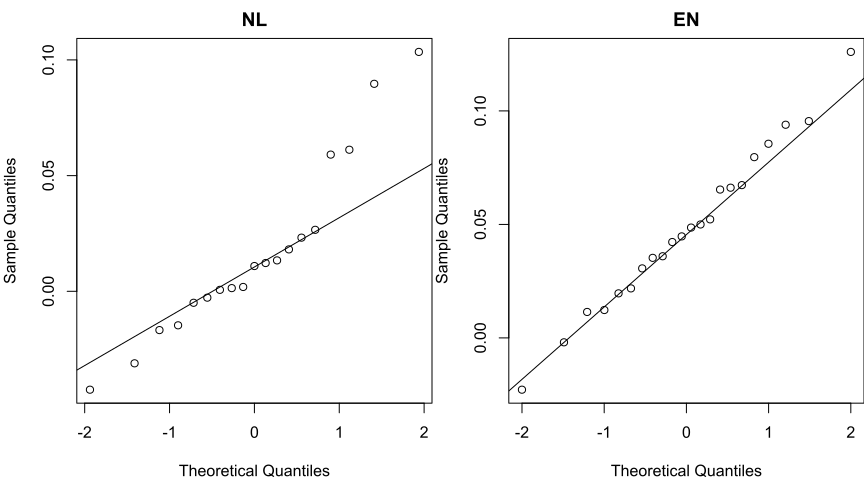## Which analysis?

• As the values in one group are not normally distributed, we used the Mann-Whitney U test to analyze the difference between the two groups
• Our $$\alpha$$-level is set at 0.05 (one-tailed)

## Analysis in R: Mann-Whitney U test

wilcox.test(diffEN$Diff, diffNL$Diff, alternative = "greater")  # 1st > 2nd?

#
#   Wilcoxon rank sum exact test
#
# data:  diffEN$Diff and diffNL$Diff
# W = 315, p-value = 0.0025
# alternative hypothesis: true location shift is greater than 0


## Conclusion of analysis

• We reject the null hypothesis, and accept the alternative hypothesis that the native English speakers show a greater tongue distinction between /t/ and /θ/ than non-native speakers (U: 315, $$p = 0.0025$$)
• If we would have incorrectly analyzed the data using the independent samples $$t$$-test, we would also have rejected the null hypothesis
• But with $$p = 0.004$$

## Effect size of Mann-Whitney U test

• Cliff's delta (or $$d$$) measures effect size of the Mann-Whitney U test
• $$|d| < 0.147$$: negl.; $$|d| < 0.33$$: small; $$|d| < 0.474$$: medium; $$|d| \geq 0.474$$: large
library(effsize)
cliff.delta(diffEN$Diff, diffNL$Diff)

#
# Cliff's Delta
#
# delta estimate: 0.50718 (large)
# 95 percent confidence interval:
#   lower   upper
# 0.13076 0.75579


## Some remarks about the Mann-Whitney U test

• Instead of the Mann-Whitney U test, an independent samples $$t$$-test of the ranks gives a $$p$$-value close to that of the Mann-Whitney U test
• E.g., A A A B A B B B B A: ranks group A = (1,2,3,5,10), ranks group B: (4,6,7,8,9)
• For our example: $$p = 0.0024$$ (Mann-Whitney U test: $$p = 0.0025$$)
• Mann-Whitney U test cannot be applied to single samples, nor paired data
• For that we use the Wilcoxon signed-rank test

## Wilcoxon signed-rank test

• Alternative to single sample or paired $$t$$-test
• Applied when data is non-normal
• However, distribution should be roughly symmetric, not skewed
• If distribution is skewed, sign test should be used
• Applicable to ordinal and numerical data

## Wilcoxon signed-rank test: hypotheses

• For paired samples:
• $$H_0$$: median of the differences $$=$$ 0
• $$H_a$$: median of the differences $$\neq$$ 0 (for two-tailed hypothesis)
• For single sample:
• $$H_0$$: distribution symmetric around $$x$$ ($$\approx \mu = x$$, due to symmetry)
• $$H_a$$: distribution non-symmetric around $$x$$ ($$\approx \mu \neq x$$)
• If $$H_0$$ rejected: results may be reported as being significantly different from $$x$$

## Wilcoxon signed-rank test: idea

• Calculate pairwise differences (single sample: with respect to single value)
• Rank the absolute differences from low to high (excluding differences of 0)
• Add the signs of the differences to the ranks
• Sum the positive ranks: $$W$$
• If $$H_0$$ true then $$W$$ close to half of the total sum of all unsigned-ranks
• $$p$$-value: obtained by assessing where $$W$$ is located in the distribution of all possible $$W$$-values for a given sample size $$n$$
• Distribution of all $$W$$-values resembles a normal distribution (for larger $$n$$)

## Distribution of $$W$$-values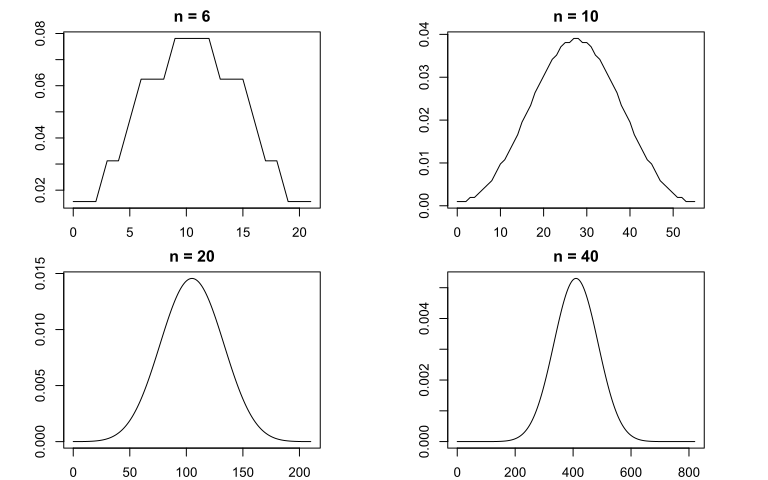## Wilcoxon signed-rank test: idea (calculations)

• Example: comparing English scores to 7.5 (only 6 cases)
english_score diff abs_diff rank signed_rank
6.78 -0.72 0.72 3 -3
5.94 -1.56 1.56 6 -6
6.18 -1.32 1.32 5 -5
8.00 0.50 0.50 2 2
7.42 -0.08 0.08 1 -1
8.63 1.13 1.13 4 4
• $$W = 2 + 4 = 6$$
• Compared to half of total sum of ranks ($$21 / 2 = 10.5$$, so quite different)

## Wilcoxon signed-rank test: additional information

• Fortunately we don't have to do this manually!
• In R: wilcox.test() (same as for Mann-Whitney U)
• Data is converted to ranks: actual values are ignored (i.e. information loss)

## Wilcoxon signed-rank test: single sample example

• Given our English proficiency data, we'd like to assess if the average English score is different from 7.5 (with $$\alpha = 0.05$$)
• $$H_0$$: $$\mu = 7.5$$
• $$H_a$$: $$\mu \neq 7.5$$
• Visualization: